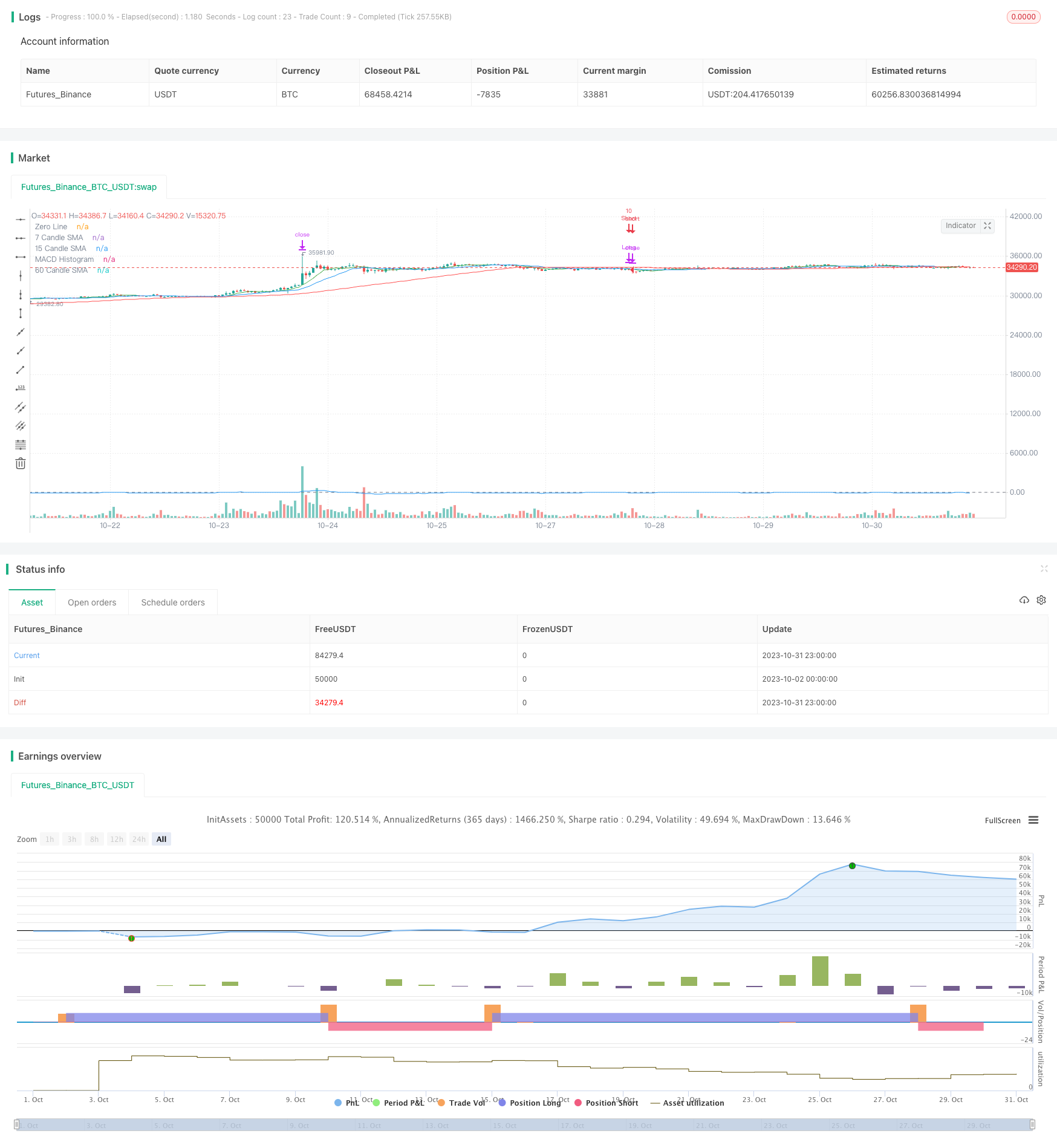# Dual Indicator Strategy

Author: ChaoZhang, Date: 2023-11-02 15:30:54
Tags:### Overview

The Dual Indicator Strategy is a quantitative trading strategy that combines Simple Moving Average (SMA) and Moving Average Convergence Divergence (MACD) indicators. By utilizing multiple technical indicators, the strategy aims to increase the accuracy of trading signals.

### Strategy Logic

The core of the Dual Indicator Strategy relies on two indicators: SMA and MACD. The strategy adopts 7-, 15- and 60-period SMAs, as well as the standard 12/26/9 MACD parameter setting.

When the 7-period SMA is above the 15- and 60-period SMAs, and the 15-period SMA is above the 60-period SMA, it is considered a bullish signal from the SMA indicator, with a probability of 0.5.

At the same time, when the MACD line crosses above the signal line, it is considered a bullish signal from the MACD indicator, also with a probability of 0.5.

When the bullish signal probabilities from the two indicators add up to 1, a long position will be opened.

Conversely, when the 7-period SMA falls below the 15- and 60-period SMA, and the 15-period SMA is below the 60-period SMA, it is considered a bearish signal from the SMA indicator, with a probability of 0.5.

Meanwhile, when the MACD line crosses below the signal line, it is considered a bearish signal from the MACD indicator, with a probability of 0.5.

When the bearish signal probabilities from the two indicators add up to 1, a short position will be opened.

In addition, the strategy adopts two different take profit points: close out 50% of the position when price rises or falls by 9%, and close out the remaining position when price rises or falls by 21%.

If a signal opposite to the current position occurs, the current position will be closed first before opening a new position based on the new signal.

The biggest advantage of the Dual Indicator Strategy is that it utilizes the strengths of both SMA and MACD indicators. SMA can effectively track price trend changes and filter out market noise, while MACD can identify short-term trend reversal opportunities. Combining the two can improve the reliability of trading signals.

In addition, adopting SMAs with different parameter settings helps discern long- to medium-term trends, while the take profit strategy locks in partial profits and controls risks.

### Risk Analysis

Some potential risks of the Dual Indicator Strategy need to be noted. As it relies solely on technical indicators, incorrect signals may occur. Also, improper take profit settings could lead to premature exit, missing major trends.

The strategy can be optimized by adjusting the SMA period parameters or incorporating additional filtering indicators to ensure more reliable signals. Take profit levels also need to be dynamically adjusted based on market volatility to sustain capturing trending moves.

### Optimization Directions

Some aspects of the Dual Indicator Strategy can be further optimized:

1. Test adding other technical indicators like RSI, Bollinger Bands for multi-indicator filtering.

2. Try machine learning algorithms to build signal judgment models using multiple variables.

3. Perform parameter tuning based on different products and timeframes.

4. Incorporate stop loss to strictly control single trade loss.

5. Optimize take profit strategy to ride sustained trends.

Through systematic backtesting and optimization, the strategy’s stability and profitability can be continuously enhanced.

### Conclusion

The Dual Indicator Strategy combines the strengths of SMA and MACD to improve signal accuracy while effectively controlling risks. With strong optimization potential and versatility, it is a robust and adaptive quantitative trading strategy. With continuous data-driven improvements, the strategy can evolve into a powerful trading system.

```/*backtest
start: 2023-10-02 00:00:00
end: 2023-11-01 00:00:00
period: 1h
basePeriod: 15m
exchanges: [{"eid":"Futures_Binance","currency":"BTC_USDT"}]
*/

//@version=5
strategy("SMA & MACD Dual Direction Strategy", shorttitle="SMDDS", overlay=true, initial_capital=1000)

// SMA settings
sma7_length = input.int(7, title="7 Candle SMA Length")
sma15_length = input.int(15, title="15 Candle SMA Length")
sma60_length = input.int(60, title="60 Candle SMA Length")

// MACD settings
fast_length = input.int(12, title="Fast Length")
slow_length = input.int(26, title="Slow Length")
signal_length = input.int(9, title="Signal Length")

// Leverage
leverage = 10

// Calculate the SMAs
sma7 = ta.sma(close, sma7_length)
sma15 = ta.sma(close, sma15_length)
sma60 = ta.sma(close, sma60_length)

// Calculate the MACD line and Signal line
[macdLine, signalLine, _] = ta.macd(close, fast_length, slow_length, signal_length)

// SMA-based Probabilities
smaBullishProb = (sma7 > sma15 and sma7 > sma60 and sma15 > sma60) ? 0.5 : 0.0
smaBearishProb = (sma7 < sma15 and sma7 < sma60 and sma15 < sma60) ? 0.5 : 0.0

// MACD-based Probabilities
macdBullishProb = ta.crossover(macdLine, signalLine) ? 0.5 : 0.0
macdBearishProb = ta.crossunder(macdLine, signalLine) ? 0.5 : 0.0

// Combined Probabilities
combinedBullishProb = smaBullishProb + macdBullishProb
combinedBearishProb = smaBearishProb + macdBearishProb

// Trade logic using `if` conditions
if combinedBullishProb == 1.0
strategy.close("Short")
strategy.entry("Long", strategy.long, qty=leverage)

if combinedBearishProb == 1.0
strategy.close("Long")
strategy.entry("Short", strategy.short, qty=leverage)

// Exit conditions based on profit points
longTargetProfit1 = close * 1.09
longTargetProfit2 = close * 1.21

shortTargetProfit1 = close * 0.91
shortTargetProfit2 = close * 0.79

strategy.exit("Long TP1", from_entry="Long", limit=longTargetProfit1, qty_percent=0.5)
strategy.exit("Long TP2", from_entry="Long", limit=longTargetProfit2)

strategy.exit("Short TP1", from_entry="Short", limit=shortTargetProfit1, qty_percent=0.5)
strategy.exit("Short TP2", from_entry="Short", limit=shortTargetProfit2)

// Visualization (optional)
plot(sma7, color=color.green, title="7 Candle SMA")
plot(sma15, color=color.blue, title="15 Candle SMA")
plot(sma60, color=color.red, title="60 Candle SMA")
hline(0, "Zero Line", color=color.gray)
plot(macdLine - signalLine, color=color.blue, title="MACD Histogram")

```

More